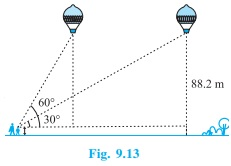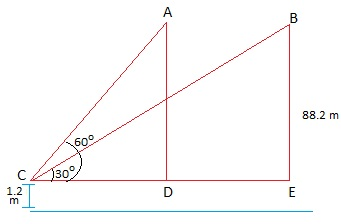# A 1.2 m tall girl spots a balloon moving with the wind in a horizontal line at a height of 88.2 m from the ground. The angle of elevation of the balloon from the eyes of the girl at any instant is 60°. After some time, the angle of elevation reduces to 30° (see Fig.) . Find the distance travelled by the balloon during the interval.On redrawing the diagram we getFrom right ΔBEC,

tan 30° = BE/CE

1/√3= 87/CE

CE = 87√3

√3= 87/CD

CD = 87/√3 = 29√3

Step 3:

DE = CE – CD = (87√3 – 29√3) = 29√3(3 – 1) = 58√3

Hence, Distance travelled by the balloon = 58√3 m.